# A New Approach to ICSE Physics Part 1 Class 9 Solutions Motion in One Dimension

## A New Approach to ICSE Physics Part 1 Class 9 Solutions Motion in One Dimension

UNIT 1

EXERCISE 1

Question 1.
What do you understand by the terms

1. rest

(i) Rest : A body is said to be at rest if it does not change its position with respect to its immediate surroundings.
For example :

(a) A chair lying in a room.
(b) A stone lying on the ground.

(ii) Motion : A body is said to be in motion if it changes its position with respect to its immediate surroundings.
For example :

(a) A car running on the road.
(b) A football moving on the ground.

Question 2.
By giving an example, prove that rest and motion are relative terms.
Rest and motion are relative terms. It means a body at rest in one situation at certain time can be in motion in another situation at the same time.
A person sitting in the compartment of a moving train is in the state of rest with respect to the surrounding of the compartment yet he is in state of motion, if he compare himself with the surroundings outside the compartment.

Question 3.
Define :

1. Scalar quantities.
2. Vector quantities. Give two differences between scalar and vector quantities.

(i) Scalar quantities : The physical quantities which are expressed in magnitude only are called scalar quantities.
For example: Mass, length, time, distance, density, energy etc.
(ii) Vector quantities : The physical quantities which are ‘ expressed in magnitude as well as direction are called vector quantities.
For example : Displacement, velocity, acceleration, momentum, force etc.
Differences between scalar and vector quantities.
SCALARS :

1. Scalars are specified by one quantity only i.e. magnitude.
2. Scalars change by change in magnitude along.
3. Scalars are written or represented by ordinary letters.
5. Mass, length, time, speed, etc. are some example of scalars.

VECTORS :

1. Vectors are specified by two quantities (i) magnitude and (ii) direction.
2. Vectors change when there is change in either magnitude or direction or both.
3. Vectors are written (shown) in bold face letters or letters having arrows heads on them.
4. Vectors are added or subtracted by using triangle law, parallelogram law or polygon law.
5. Displacement, velocity acceleration are some examples of vectors.

Question 4.
Pick out the scalar and vector quantities from the following list:

1. mass
2. density
3. displacement
4. distance
5. momentum
6. acceleration
7. temperature
8. time

1. Scalar quantities : Mass, density, distance, temperature, time.
2. Vector quantities : Displacement, momentum, acceleration.

Question 5.
Define :
(i) Speed (ii) Velocity. Give two differences between speed and velocity.
(i) Speed : The distance covered by a body in a unit time is called its speed. It is also defined as the rate of change position of a body in any direction.
speed = distance / time
(ii) Velocity : “Rate of change of displacement with time” is called velocity, or “The time rate of change of displacement of an object” is called the velocity.
V = distance/time
Differences between speed and velocity
Speed :

1. The rate of change of position of a body in any direction is known as its speed.
2. It is a scalar quantity.
3. It can be positive or zero.

Velocity :

1. The rate of change of position of a body in a particular direction is known velocity.
2. It is a vector quantity.
3. It can be positive, negative or zero.

Question 6.
Define (i) Distance (ii) Displacement. Give two differences between displacement and distance.
(i) Distance : The length of path travelled by a body in certain interval of time is called distance.
(ii) Displacement : of an object between two points is the shortest distance between these two points.
“It is the unique path which can take the body from its initial to final position.”
Differences between displacement and distance.
Distance :

1. It is a scalar quantity.
2. Distance travelled is always positive.
3. The distance travelled by a moving body is the actual length of path.
4. Distance travelled is always greater than or equal to the displacement.
5.Displacement :

1. It is a vector quantity.
2. Displacement may be positive negative or zero.
3. The displacement of a body is the shortest distance between the initial and final positions of the body.
4. Displacement is always less than or equal to the distance travelled.
5.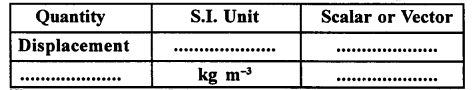Question 7.
By giving one example each, define

1. variable velocity.
2. average velocity.
3. uniform velocity.

(i) Variable velocity : When a body covers unequal distances in equal intervals of time in a specified direction, the body is said to be moving with a variable velocity.
Example : A rotating fan at a constant speed has variable velocity, because of continuous change in direction.
(ii) Average velocity: The ratio of the total distance travelled in a specified direction to the total time taken by the body to travel the distance is called average velocity.
Example : If you walk to a campsite 1 km away and then back to your starting point with in 1 hour, then your average velocity will be zero because your initial and final position is same.
(iii) Uniform velocity : When a body covers equal distances in equal intervals of time (however small may be the time interval) in a specified direction, the body is laid to be moving with uniform velocity.
Example : A car moving on a straight road with constant speed has uniform velocity.

Question 8.
What do you understand by the term acceleration? When is the acceleration

1. positive
2. negative?

• Acceleration : “Rate of change of velocity with respect to time” is called acceleration. S.I. unit of acceleration is m s-2.
• Positive acceleration : When velocity of a body increases with time, then acceleration of the body is said to be positive acceleration.
• Negative acceleration : When the velocity of a body decreases with time, then acceleration of the body is said to be negative acceleration.

Question 9.
Define the term acceleration due to gravity. State its value in C.GS. as well as in S.I. system. When is acceleration due to gravity

1. positive
2. negative?

Acceleration due to gravity : The acceleration of a freely falling body, under the action of gravity of earth, is called acceleration due to gravity. It is represented by ‘g’.
In S.I. system, g = 9.8 ms-2 ; In C.GS. system, g = 980 cms-2 If a body falls towards the earth, then value of acceleration due to gravity is positive.
If the body rises vertically upwards, then value of acceleration due to gravity is negative.

Question 10.
Give an example of a body which covers a certain distance, but its displacement is zero.
Yes, displacement of a body can be zero even if the distance covered by it is not zero. For example, if a body moves in a circle, then displacement of the body in one rotation is zero but the distance covered by it in one roation = 2πr, where r is the radius of the circle in which the body is moving.

Question 11.
Give an example of an accelerated body, moving with a uniform speed.
When a body moves over a circular path with constant speed, then body is said to be accelerated. Acceleration is produced in the body due to continuous change in its direction.

Question 12.
What is the relation between distance and time when

1. body is moving with uniform velocity
2. body is moving with variable velocity?

1. When body moves with uniform velocity, then body covers equal distance in equal interval of time in a specified direction.
2. When a body moves with variable velocity, then body covers unequal distance in equal interval of time in a specified direction.

Question 13.
(a) Distinguish between scalar and vector quantities,
(b) State whether following are scalar or vector quantities

1. speed
2. force
3. acceleration
4. energy

(a) See Q. No. 3 of Exercise-1
(b)

1. Speed is a scalar quantity because it has magnitude and no direction.
2. Force is a vector quantity because it has both magnitude as well as direction.
3. Acceleration is a vector quantity.
4. Energy is a scalar quantity because it has magnitude and no direction.

Question 14.
Copy the following table and fill in the blank spaces.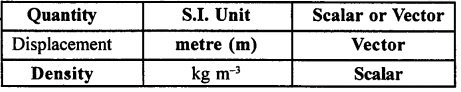Question 15.
Draw a diagram to show the motion of a body whose speed remains constant, but velocity changes continuously.
A body moving in a circle with constant speed but variable velocity as the velocity of the body at any point is along the tangent to the circle at that point as shown in the figure.Practice Problems 1

Question 1.
A car covers 90 km in 1 1/2 hours towards east. Calculate
(i) displacement of car,
(ii) its velocity in (a) kmh-1
(b) ms-1.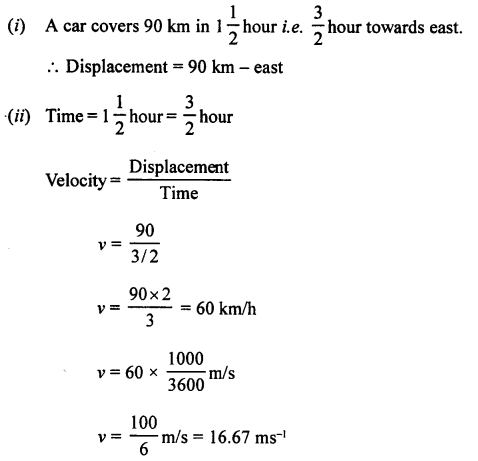Question 2.
A race horse runs straight towards north and covers 540 m in one minute. Calculate (i) displacement of horse, (ii) its velocity in (a) ms-1 (b) kmh-1.
A race horse runs straight towards north and covers 540 m in one minute.

1. Displacement = 540 m – north
2. Time = 1 minute = 60 s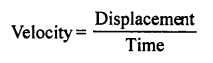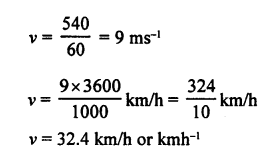Practice Problems 2

Question 1.
The change in velocity of a motor bike is 54 kmlr1 in one minute. Calculate its acceleration in (a) ms-2 (b) kmh-2.Question 2.
A speeding car changes its velocity from 108 kmh-1 to 36 kmh-1 in 4 s. Calculate its deceleration in

1. ms-2
2. kmh-2.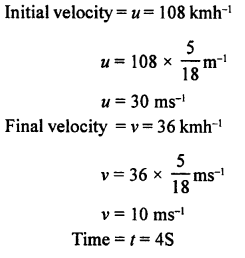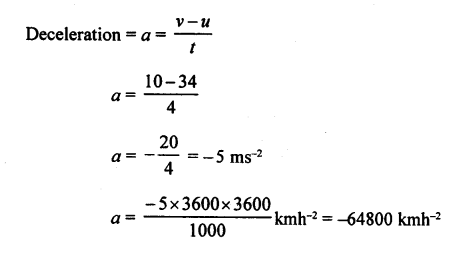UNIT II
EXERCISE 2

(A) Objective Questions

Multiple choice Questions. Select the correct option.

Question 1.
A graph is a straight line parallel to the time axis in a distance – time graph. From the graph, it implies :
(a) body is stationary
(b) body is moving with a uniform speed
(c) body is moving with a variable speed
(d) none of these
(a) body is stationary

Question 2.
The slope of a displacement – time graph represents :
(a) uniform speed
(b) non-uniform speed
(c) uniform velocity
(d) uniform a acceleration
(c) uniform velocity

Question 3.
A body dropped from the top of a tower reaches the ground in 4s. Height of the tower is
(a) 39.2 m
(b) 44.1 m
(c) 58.8 m
(d) 78.4 m
(d) 78.4 m
Explanation :
Initial velocity = u = 0
Time = t = 4s
As body falls downards,
so acceleration = a = + g= a = + 9.8 ms-2
(Distance) S = ut + 1/2 at
S = (0) (4) + 1/2 × 9.8 × (4)3
s = 0 + 1/2 × 9.8 × 16
S = 9.8 × 8 = 78.4 m

Question 4.
The speed of a car reduces from 15 m/s to 5 m/s over a displacement of 10 m.
The uniform acceleration of the car is :
(a) -10 m/s2
(b) +10 m/s2
(c) 2 m/s2
(d) 0.5 m/s2
(a) -10 m/s2
Explanation :
Initial velocity = u = 15 m/s
Final velocity = v = 5 m/s
Displacement = S = 10 m
Acceleration = a = ?
v2 – u2 = 2aS ; (5)2 – (15)2 = 2a (10)
25 – 225 = 20a
a = -220/20 = -10 ms-2

Question 5.
A body projected vertically up with a velocity 10 m/s reaches a height of 20 m. If it is projected with a velocity of 20 m/s, then the maximum height reached by the body is :
(a) 20 m
(b) 10 m
(c) 80 m
(d) 40 m
(b) 10 m
Explanation :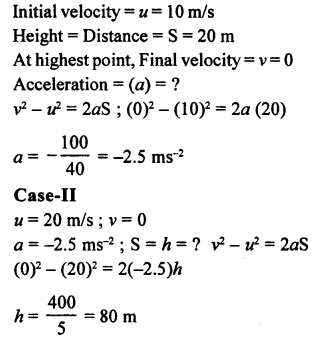Question 6.
What does the area of an acceleration – time graph represent?
(a) Uniform velocity
(b) Displacement
(c) Distance
(d) Variable velocity
(a) Uniform velocity

Question 7.
A driver applies brakes when he sees a child on the railway track, the speed of the train reduces from 54 km/h to 18 km/h in 5 s. What is the distance travelled by the train during this interval of time?
(a) 52 m
(b) 50 m
(c) 25 m
(d) 80 m
(b) 50 mQuestion 8.
In velocity time graph, the acceleration is
(a) – 4 m/s2
(b) 4 m/s2
(c) 10 m/s2
(d) zero(a) -4 m/s2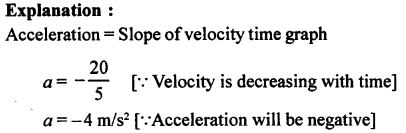Question 9.
The distance covered in adjoining velocity – time graph is :
(a) 25 m
(b) 40 m
(c) 50 m
(d) 45 m(c) 50 m
Explanation :
Distance covered = Area under velocity – time graph
=1/2 × base × height
a= 1/2 × 5 × 20 = 50 m

Question 10.
At the maximum height, a body thrown vertically upwards has :
(a) velocity not zero but acceleration zero.
(b) acceleration not zero but velocity zero.
(c) both acceleration and velocity are zero.
(d) both acceleration and velocity are not zero.
(b) acceleration not zero but velocity zero.

(B) Subjective Questions

Question 1.
Draw displacement – time graphs f.or the following situations :

1. When a body is stationary.
2. When a body is moving with uniform velocity.
3. When a body is moving with variable velocity.

(i) Displacement – time graph when body is stationary.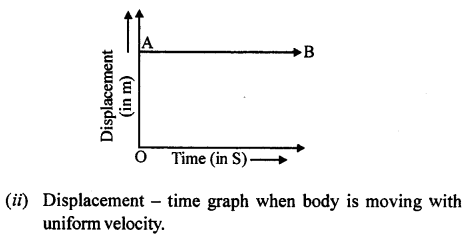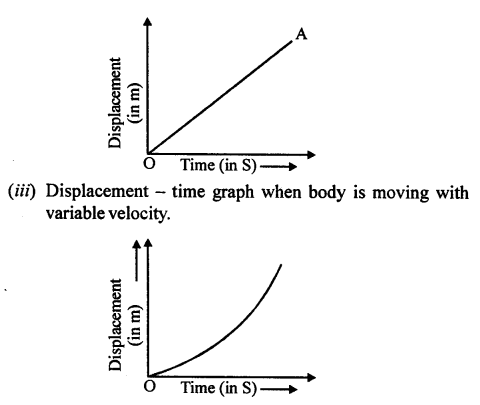Question 2.
Draw velocity – time graphs for the following situations :

1. When a body, is moving with uniform velocity.
2. When a body is moving with variable velocity, but uniform acceleration.
3. When a body is moving with variable velocity, but uniform retardation.
4. When a body is moving with variable velocity and variable acceleration.

Following are the velocity – time graph :
(i) When a body is moving with uniform velocity : Then velocity time graph is AB is a straight line parallel to time axis.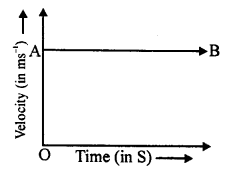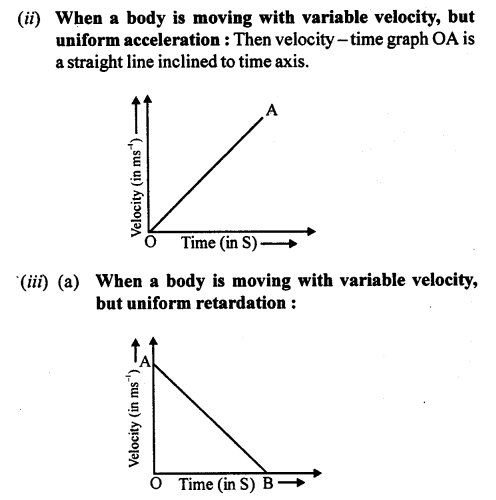Question 3.
How can you find the following?

1. Velocity from a displacement – time graph.
2. Acceleration from velocity – time graph.
3. Displacement from velocity – time graph.
4. Velocity from acceleration – time graph.

(i) Velocity from a displacement – time graph : Displacement time graph for uniform motion is a straight line (OP) inclined to time axis. Take any two points A and B on this graph OP. From A and B, draw perpendiculars on time axis as well as displacement axis.
So, by knowing the slope of displacement – time graph. We can find the velocity of the body.(ii) Acceleration from velocity – time graph : Velocity – time graph for uniform motion is a straight line (OP) inclined to time axis.Take any two points A and B on this graph. From A and B draw perpendicular on time axis as well as velocity axis in such a way thatSo, by knowing the slope of velocity -time graph for uniform motion, we can find the acceleration of the body.
(iii) Displacement from velocity-time graph: Displacement covered by a body is equal to the area under velocity – time graph. When object moves with a uniform velocity, then velocity – time graph is a straight line (PQ) parallel to time axis.(iv) Velocity from acceleration – time graph : Area under the acceleration – time graph gives the velocity of the body. When the body moves with variable velocity but uniform acceleration, then acceleration – time graph is a straight line (PQ) parallel to time axis.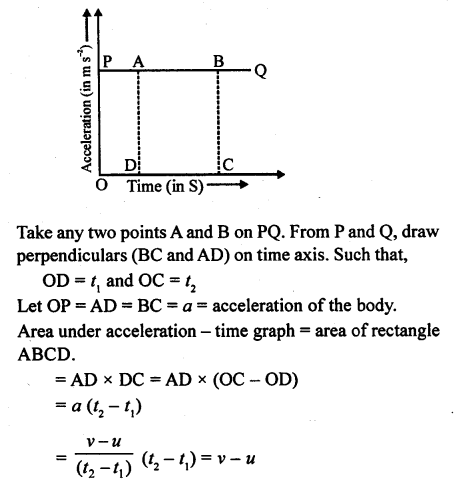If initial velocity of the body = u = 0 Then area under acceleration – time graph = v – 0 = v = velocity of the body.

Question 4.
What do you understand by the term acceleration due to gravity? What is its value in C.G.S. and S.I. systems?
Acceleration due to gravity : The acceleration of a freely falling body, under the action of gravity of earth, is called acceleration due to gravity. It is represented by ‘g’.
In S.I. system, g = 9.8 ms-2; In C.G.S. system, g = 980 cms-2
If a body falls towards the earth, then value of acceleration due to gravity is positive.
If the body rises vertically upwards, then value of acceleration due to gravity is negative.

Question 5.
Can you suggest about the kind of motion of a body from the following distance – time graphs?(a) Distance – time graph in figure (a) shows that body is stationary.
(b) Distance – time graph figure (b) shows that body is in uniform motion.
(c) Distance – time graph in figure (c) shows that initially body is in uniform motion i.e. it covers equal distance in equal intervals of time.
From O to A, body is in uniform motion and from A to B, body is at rest.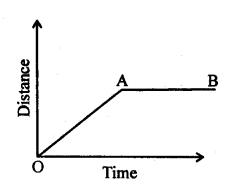Question 6.
Can you suggest real life examples about the motion of a body from the following velocity – time graphs?(a) A car running on a road at constant velocity. Then v-f graph will be same as shown by (a).
(b) Figure (b) shows that car is moving with uniform acceleration. i.e. when velocity of car increases equally in equal intervals of time.
(c) Suppose a train starts from rest and accelerates uniformly for some time and then moves with a constant velocity for certain time interval. Then on applying the brakes, train is uniformly retarded and comes to rest after sometime in this situation, velocity – time graph of the train will be same as showin in figure (c).
(d) When you come home at activa from market and stops in front of your home. Then velocity of active decreases with time and its v-f graph will be same as shown on figure (d).
(e) A ball is thrown vertically upward and it returns back to earth after sometime. In this situation, graph will same as that shown in figure (e).

Question 7.
Diagram shows a velocity – time graph for a car starting from rest. The graph has three section AB, BC and CD.1. From a study of this graph, state how the distance travelled in any section is determined.
2. Compare the distance travelled in section BC with the distance travelled in section AB.
3. In which section, car has a zero acceleration?
4. Is the magnitude of acceleration higher or lower than that of retardation ? Give a reason.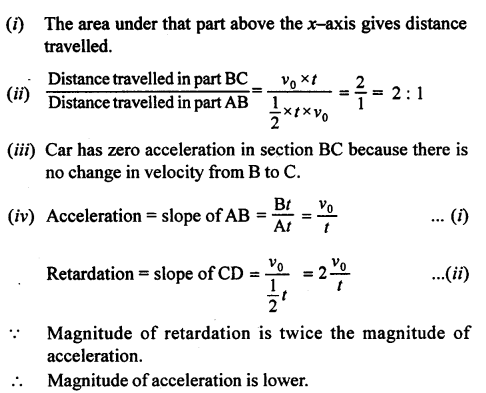Question 8.
Write down the type of motion of a body along the A – O – B in each of the following distance – time graphs.1. Body is stationary.
2. Body is in uniform motion i.e. it covers equal distance in equal intervals of time.
3. From A to O, body is in uniform motion having positive slope and from O to B, body is in uniform motion having negative slope.

Practice Problems 1

Question 1.
From the displacement – time graph shown given below calculate : –

1. Average velocity in first three seconds.
2. Displacement from initial position at the end of 13 s.
3. Time after which the body is at the initial position,
4. Average velocity after 8 s.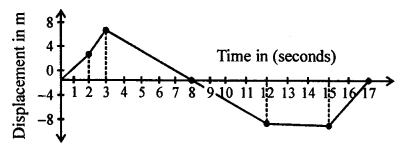(i) In first three seconds
Total displacement = 8m
Total time = 3 s
Average velocity = Total displacement / Totaltime = 8/3
= 2.67 ms-1
(ii) Displacement from initial position at the end of 13s=-8m.
(iii) Body is at the initial position after 8s and 17s.
(iv) Average velocity after 8s is zero because after 8s, displacement is zero.

Question 2.
From the displacement – time graph shown given below calculate :

1. Velocity between 0 – 2 s.
2. Velocity between 8 s – 12 s.
3. Average velocity between 5 s – 12 s.Practice Problems 2

Question 1.
A train starting from rest, picks up a speed of 20 ms-1 in 200 s. It continues to move at the same rate for next 500s, and is then brought to rest in another 100 s.
(i) Plot a speed-time graph.
(ii) From graph calculate
(a) uniform rate of acceleration
(b) uniform rate of retardation
(c) total distance covered before stopping
(d) average speed.
(i)Question 2.
A ball is thrown up vertically, and returns back to thrower in 6 s. Assuming there is no air friction, plot a graph between velocity and time. From the graph calculate

1. deceleration
2. acceleration
3. total distance covered by ball
4. average velocity.

A ball is thrown up vertically, and returns to thrower in 6s. It means ball takes 3s to reach the highest point and 3s to reach the earth from highest point.Question 3.
A racing car is moving with a velocity of 50 m/s. On
applying brakes, it is uniformly retarded and comes to rest in 20 seconds. Calculate its acceleration.Question 4.
A body falls freely downward from a certain height. Show graphically the relation between the distance fallen and square of time. How will you determine ‘g’ from the graph?
For a freely falling body, the displacement is directly proportional to the square of time. By knowing the slope of displacement Vs. square of time graph, we can find the acceleration due to gravity.

Question 5.
A body at rest is thrown downward from the top of tower. Draw a distance – time graph of its free fall under gravity during first 3 seconds. Show your table of values starting t = 0 with an interval of 1 second, (g = 10 ms-2).
Initial velocity = M = 0
Acceleration = a = +g = 10 ms-2
when t = Is, then distance travelled (S,) is given by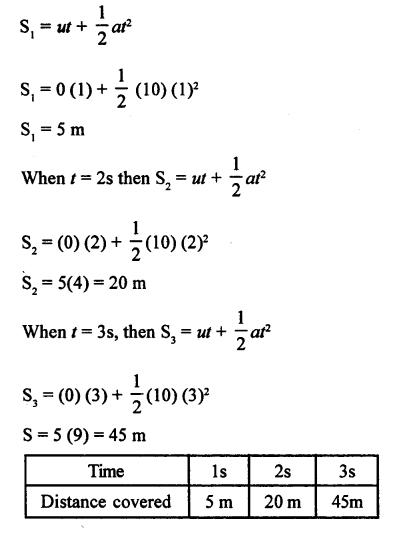Practice Problems 3

Question 1.
From the diagram given below, calculate

1. acceleration
2. deceleration
3. distance covered by body.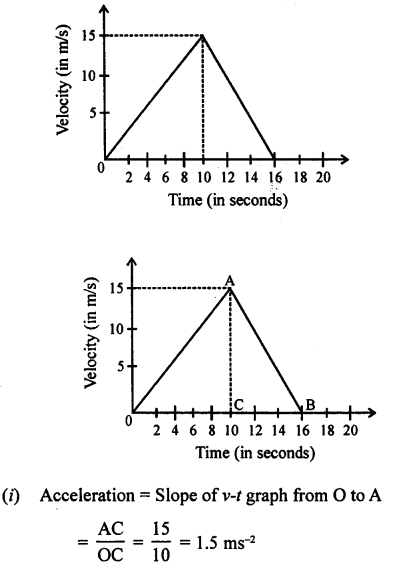= 5 × 15 + 3 × 15
= 75 + 45 = 120 m

Question 2.
From the velocity – time graph given below, calculate :

1. Acceleration in the region AB.
2. Deceleration in region BC.
3. Distance covered in the region ABCE.
4. Average velocity in region CED.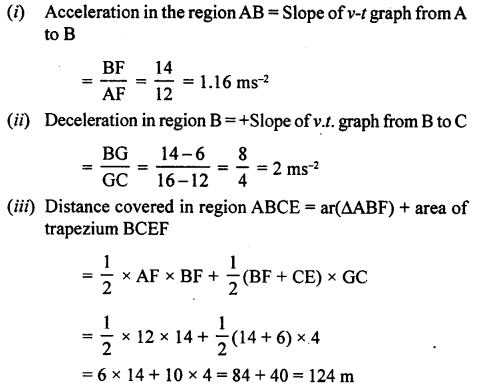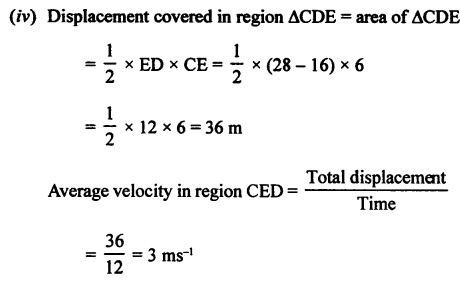Practice Problem 4

Question 1.
Diagram given below shows velocity – time graphs of car P and Q, starting from same place and in same direction. Calculate :

1. Acceleration of car P.
2. Acceleration of car Q between 2 s – 5 s.
3. At what time intervals both cars have same velocity?
4. Which car is ahead after 10 s and by how much?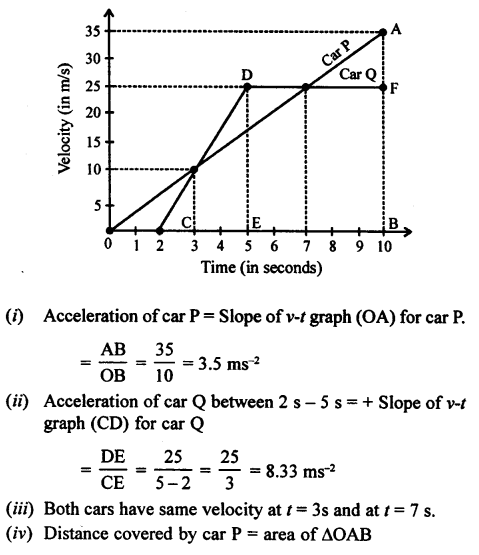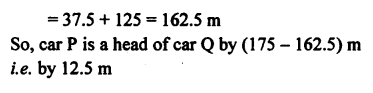UNIT III

Practice Problems 1

Question 1.
A motor bike, initially at rest, picks up a velocity of 72 kmh-1 over a distance of 40 m. Calculate

1. acceleration
2. time in which it picks up above velocity.

Initial velocity = u = 0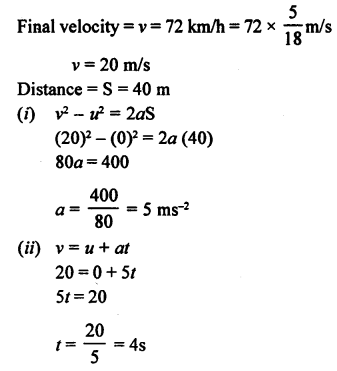Question 2.
A cyclist driving at 5 mr-1, picks a velocity of 10 ms-1, over a distance of 50 m. Calculate

1. acceleration
2. time in which the cyclist picks up above velocity.

Initial velocity = u = 5 ms-1
Final velocity = v = 10 ms-1
Distance = S =50 m
(i) v2 – u = 2aS
(10)2 – (5)2 = 2a (50)
100a = 100—25=75Practice Problems 2

Question 1.
An aeroplane lands at 216 kmh-1 and stops after covering a runway of 2 m. Calculate the acceleration and the time, in which it comes to rest.
Initial velocity = u = 216 kmh-1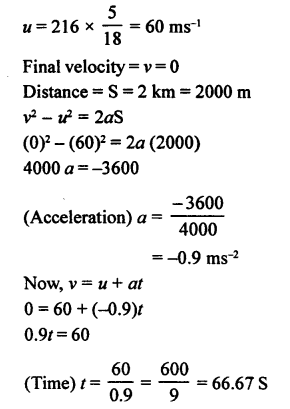Question 2.
A truck running at 90 kmh-1, is brought to rest over a distance of 25 m. Calculate the retardation and time for which brakes are applied.Practice Problems 3

Question 1.
A racing car, initially at rest, picks up a velocity of 180 kmh-1 in 4.5 s. Calculate

1. acceleration
2. distance covered by car.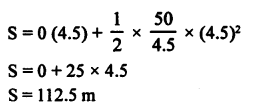Question 2.
A motor bike running at 5 ms-1, picks up a velocity of 30 ms-1 in 5s. Calculate

1. acceleration
2. distance covered during acceleration.Practice Problems 4

Question 1.
A motor bike running at 90 kmh-1 is slowed down to 18 kmh-1 in 2.5 s. Calculate

1. acceleration
2. distance covered during slow down.

Initial velocity of motor bike = u = 90 kmh-1
= u = 90 x 5/18 ms-1 = 25 ms-1
Final velocity of motor bike = v = 18 kmh-1Question 2.
A cyclist driving at 36 kmh-1 stops his motion in 2 s, by the application of brakes. Calculate

1. retardation
2. distance covered during the application of brakes.Practice Problems 5

Question 1.
A motor bike running at 90 kmh-1, is slowed down to 54 kmh-1 by the application of brakes, over a distance of 40 m. If the brakes are applied with the same force, calculate

1. total time in which bike comes to rest
2. total distance travelled by bike.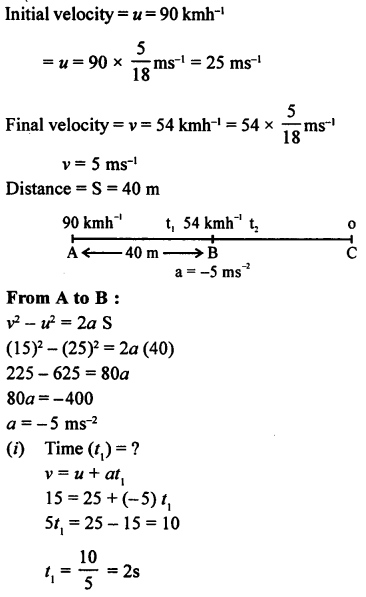Total distance covered from A to C = 40 + 22.5 = 62.5
Total time taken from A to C = t1 + t2 + = + 3 = 5s

Question 2.
A motor car slows down from 72 kmh-1 to 36 kmh-1 over at distance of 25 m. If the brakes are applied with the same force
calculate

1. total time in which car comes to rest
2. distance travelled by it.Practice Problems 6

Question 1.
A packet is dropped from a stationary helicopter, hovering at a height ‘h’ from ground level, reaches ground in 12s. Calculate

1. value of  h
2. final velocity of packet on reaching ground. (Take g = 9.8 ms 2)Question 2.
A boy drops a stone from a cliff, reaches the ground in 8 seconds. Calculate

1. final velocity of stone
2. height of cliff. (Take g = 9.8 ms’2)

Initial velocity = u = 0
Time = t = 8s
Final velocity = v = ?
Height of cliff = h = ?
A cceleration = a = + g = + 9.8 ms-1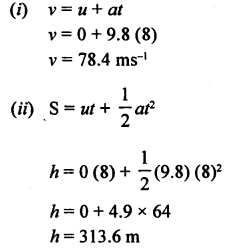Practice Problems 7

Question 1.
A stone thrown vertically upwards, takes 3 s to attain maximum height. Calcualte

1. initial velocity of the stone
2. maximum height attained by the stone. (Take g = 9.8 ms-2)Question 2.
A stone thrown vertically upwards, takes 4 s to return to thrower. Calculate
(i) initial velocity of the stone
(ii) maximum height attained by stone. (Take g = 10 ms-2)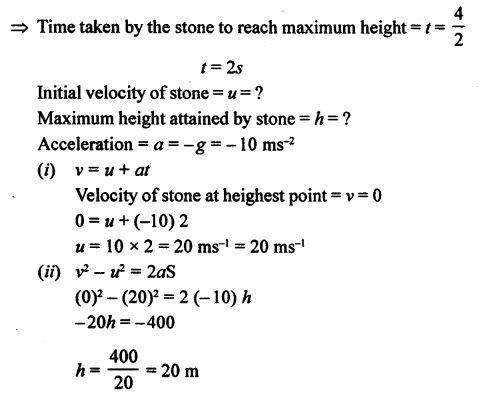Practice Problems 8

Question 1.
A spaceship is moving in space with a velocity of 50 kms-1. Its engine fires for 10 s, such that its velocity increases to 60 kms-1. Calculate the total distance travelled by
spaceship in 1/2 minute, from the time of firing its engine
Initial velocity of spaceship = u = 50 kms-1
u- 50 x 1000 ms-1
u = 50000 ms-1
Time = t= 10s
Final velocity of spaceship = v = 60 kms-1
v = 60 x 1000 ms-1 = 6000 ms-1
Acceleration = a = ?Question 2.
A spaceship is moving in space with a velocity of 60 kms-1. It fires its retro engines for 20 second and velocity is reduced to 55 kms-1. Calculate the distance travelled by the spaceship in 40 s, from the time of firing of the retro- rockets.